# MA: COST ESTIMATION AND BEHAVIOUR

##### In this unit, we shall look at the presentation of financial information for decision making and cost control. We shall be considering how the management accountant can be of assistance in providing answers to question about the consequences of following a particular action.

COST ESTIMATION AND BEHAVIOUR

IMPORTANCE OF COST BEHAVIOUR

The study of the behaviour of costs has to do with the classification of costs which form the basis for the prediction of the future level of activity Costs can be categorised into: Variable costs, fixed costs and semi-variable costs.

The Level of Activity.

The volume or level of activity constitutes the main basis for forecasting costs especially where changes or future changes are to measured. The level of activity can be viewed severally, for example, tonnage produced, standard or predetermined hours required, bed space occupied, grammage of stock used, kilometres covered per hour, passenger per bus, etc.

VARIABLE COST

A variable cost (VC) is defined as:
“A cost which varies with the measure of activity”. (CIMA)

Variable Cost Behaviour

Variable cost can be analysed into two main groups: Linear and non-linear or curvilinear.

(a) Linear Variable Cost

This is the easiest way of sharing the relationship between total variable cost and output as a straight line graph thus: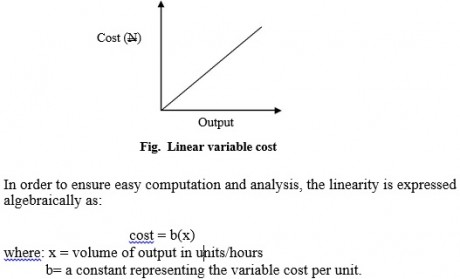Illustration(b) Non-Linear or Curvilinear Variable Costs
The non-linear cost curve establishes the relationship between output and variable cost as that of a curved line on a graph. This can be shown in two ways, thus:

convex – where each extra unit of output causes a less than proportionate increase in cost

concave – where each extra unit of output causes a more than proportionate increase in cost.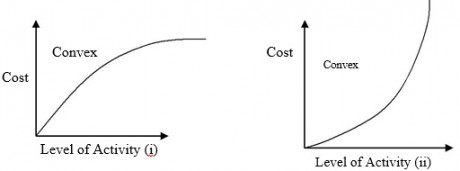A situation whereby there is an additional increase in the input of raw materials in the production process, brings about a proportionate decrease in output will result in a convex cost function.

Non-Linear Variable Costs – the Parabola

A curve is referred to as a parabola in a situation whereby changes in the level of output brings about a uniform change in the gradient of the cost function. It can be shown algebraically thus:ILLUSTRATION

Analysis of cost and activity records for a project show that the variable cost can be accurately represented by the function: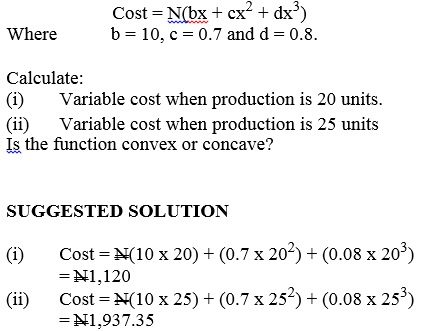It will be seen that a slight increase in activity from 20 to 25 units results in almost doubling of variable cost. This shows that there is a more than proportionate increase in the unit cost of extra production. Therefore, the function is concave.

FIXED COST

A fixed cost is defined as:

“A cost which is incurred for an accounting period, and which, within certain output or turnover limits tends to be unaffected by fluctuations in the level of activity (output or turnover)”(CIMA)’.

A fixed cost can also be referred to as a period cost.

Since fixed costs are a function of time, they do not respond to changes in activity levels. Therefore, changes in cost is not related to changes in the volume of activity within a given range of activity Fixed cost increased or stepped up only after a range of activity and not at every level of activity.

Even though, fixed costs can be depicted on a graph, it can also be shown algebraically as: = ‘a’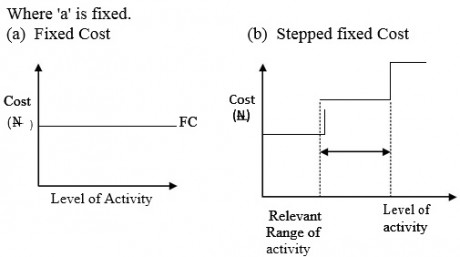Fixed cost and Stepped fixed cost

It means that at any level of activity, the fixed cost remained the same.

SEMI-VARIABLE COSTS

This type of cost can be defined as;

“A cost containing both fixed and variable components and which is thus partly affected by a change in the level of activity”, (CIMA).

Examples of semi-variable cost are: NEPA bill, NITEL telephone bills, Water rate, and some GSM operators bills.Semi-variable cost

Semi-variable costs can also be expressed algebraically by bringing together the expressions for variable cost and fixed cost thus:

Linear Semi-variable cost = a + bx:

where ‘a’ represents fixed cost, b represent the unit variable cost and x represents the level of activity.

Non-linear semi variable cost = a + bx + cx2 +dx3+… + pxn is expressed in 6.1.2 (b)

ILLUSTRATION

A breakdown of maintenance packaging department of Uzuh Limited costs shows that there is a fixed element of N5,000 per month and a variable element related to hours amounting to N6.00 per machine hour.

What is the expected cost for a month when the planned activity level is
(i) 2,500 machine hours,
(ii) 3,000 machine hours?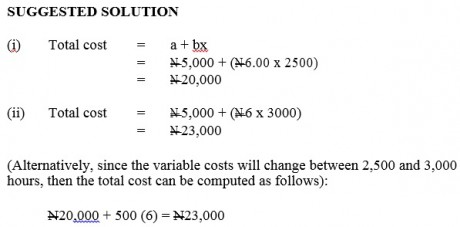Note:

In another way, the semi-variable costs can be referred to as semi-fixed and mixed costs.

COST ESTIMATION

Cost estimation is a term used to describe the measurement of historical cost with a view to helping in the prediction of future costs for management decision making, i.e. historic information is analysed to provide estimates on which to base future expectations.

Mixed costs can be separated into their fixed and variable elements, using a variety of techniques. Some techniques are more sophisticated than others, and, therefore, likely to be more reliable, but in practice, the simpler techniques are more commonly found.

Techniques Used in Prediction / Estimation

(a) The account classification method / account analysis techniques
(b) The high – low method or the Range method.

(c) Scatter graph.
(d) Regression Analysis.

(a) Account Classification Method

This is the examination of each item of expenses which involves classifying them into variable, and fixed cost using the subjective judgement of the Accountant.

(i) It is fast and inexpensive.

(ii) It is simple and easy to understand.

(iii) It can be revised regularly to account for changes in cost structure or cost classification.

(i) The initial classification has considerable subjective element.

(ii) It relies on a single observation to determine the cost function.
(iii) It is based on past costs.

(iv) Separation of semi-variable cost into its fixed and variable component is arbitrary.

(b) High-Low Method/Range Method

By this method, a check is made of historic costs in previous accounting periods, and the costs in the two particular periods are selected for cost estimation, namely:

(i) the period with the highest volume of output;

(ii) the period with the lowest volume of output (Note: the periods with highest/lowest output may not be the periods of highest or lowest cost).

Where inflation makes the costs in each period uncomparable, costs should be adjusted to the same level by means of a price level index.

The difference between the total cost of the high output and the total cost of the low output will be the variable cost of the difference in output level.

ILLUSTRATION

The costs of operating the maintenance department of a computer manufacturer, for the last five months have been as follows: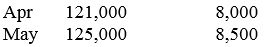What cost should be expected in month 6 when output is expected to be 10,000 standard hours? Ignore inflation.

SUGGESTED SOLUTION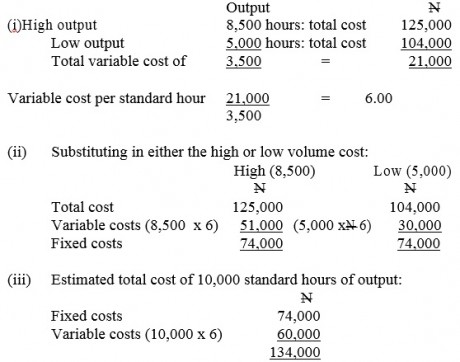Advantages of High and Low Method
(i) It is relatively simple to operate.

(ii) It is a quick and an inexpensive method of determining the underlying relationship of cost and level of activity.

(iii) It is not subjective.

Disadvantages of High and Low Method
(i) It ignores any information between the two extreme observations.
(ii) When the extreme points are not typical, the function calculated will reflect an abnormal rather than normal cost function (i.e. a situation whereby there is no relationship between the level of activity and the costs).

(iii) It is not fully represented, because it does not make use of the whole of the available data.

The Effect of Inflation

When two or more years are compared to determine the variable cost and fixed cost for estimation, it is likely that the price -index might have changed hence there is need to adjust for the effect of inflation before arriving at the variable cost and fixed cost for prediction purposes.

(c) Scatter Graph Technique

This is a visual technique which co-ordinates the cost and the level of activity of historical records for a period of time and are plotted on a graph. At the point of interception with the cost axis, the fixed cost emerges, the shape of the line represents the rate of change of cost with activity level which is variable cost.(d) Regression Analysis Technique

This is a statistical technique that is based on historical data. If it involves one independent variable, it is called “Simple Regression; whereas when the use of more than one independent variables is involved, it is referred to as “Multiple Regression”. However, the least square regression analysis is determined by the formulae as follows;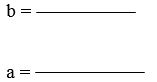x = independent variable y = dependent variable

= Summation
n = is the number of pairs of data for x and y

(i) Line of best fit can be easily recognized and could be extended through the use of multiple regression analysis.

(ii) It uses the whole data, unlike the accounts classification and high and low methods.
(iii) It assists in the use of computers and electronic calculation.

(i) A reasonable number of observation is required.

(ii) The elimination of non-random variables can reduce the available data and frustrate any attempt to fix the curve statistically to the observation.

(iii) A true relationship may not be linear, it may be curve-linear.

CONCLUSIONS

Costs frequently do not behave in regular manner and a cost function may be linear, curve-linear or stepped at different activity levels. Therefore, the ability to forecast costs is a vital part of supplying information for planning and decision making.

There are basically four ways to cost prediction or estimation. These are account analysis, scatter-graph, high and low (range method) and linear regression analysis method.

SEE ALL
•YOU